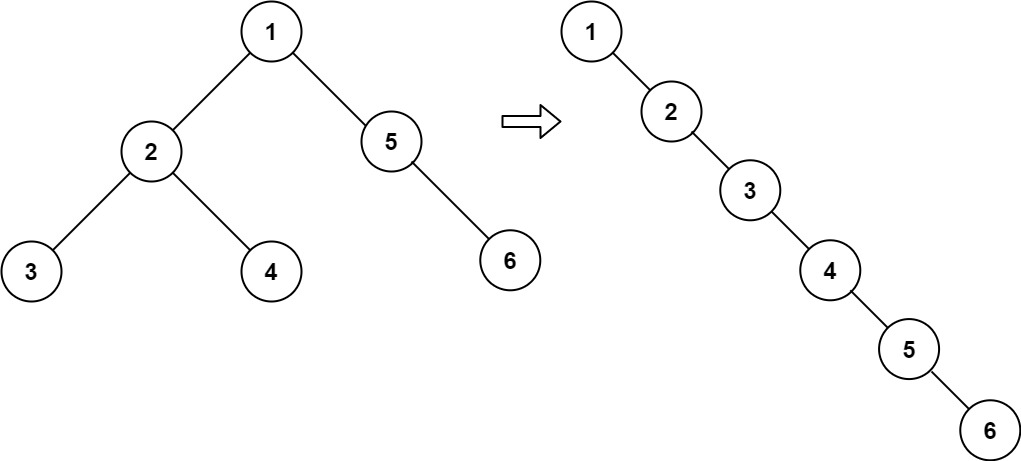## 114. Flatten Binary Tree to Linked List

Given the `root` of a binary tree, flatten the tree into a "linked list":

• The "linked list" should use the same `TreeNode` class where the `right` child pointer points to the next node in the list and the `left` child pointer is always `null`.
• The "linked list" should be in the same order as a pre-order traversal of the binary tree.

Example 1:```Input: root = [1,2,5,3,4,null,6]
Output: [1,null,2,null,3,null,4,null,5,null,6]
```

Example 2:

```Input: root = []
Output: []
```

Example 3:

```Input: root = 
Output: 
```

Constraints:

• The number of nodes in the tree is in the range `[0, 2000]`.
• `-100 <= Node.val <= 100`

Follow up: Can you flatten the tree in-place (with `O(1)` extra space)?

## Rust Solution

``````struct Solution;
use rustgym_util::*;

trait Postorder {
}

fn postorder(self, prev: &mut TreeLink) {
if let Some(node) = self {
let left = node.borrow_mut().left.take();
let right = node.borrow_mut().right.take();
right.postorder(prev);
left.postorder(prev);
node.borrow_mut().right = prev.take();
*prev = Some(node);
}
}
}

impl Solution {
let mut prev: TreeLink = None;
root.take().postorder(&mut prev);
*root = prev;
}
}

#[test]
fn test() {
let mut root = tree!(1, tree!(2, tree!(3), tree!(4)), tree!(5, None, tree!(6)));
let res = tree!(
1,
None,
tree!(
2,
None,
tree!(3, None, tree!(4, None, tree!(5, None, tree!(6))))
)
);
Solution::flatten(&mut root);
assert_eq!(root, res);
}
``````

Having problems with this solution? Click here to submit an issue on github.